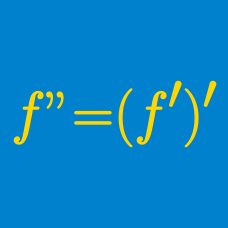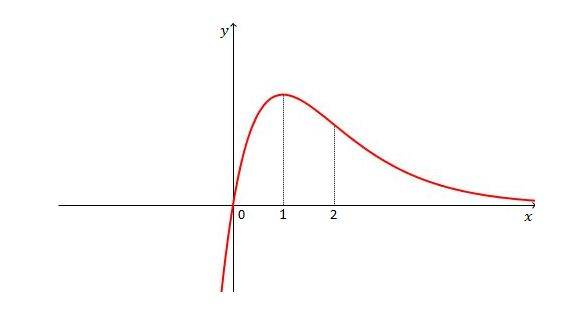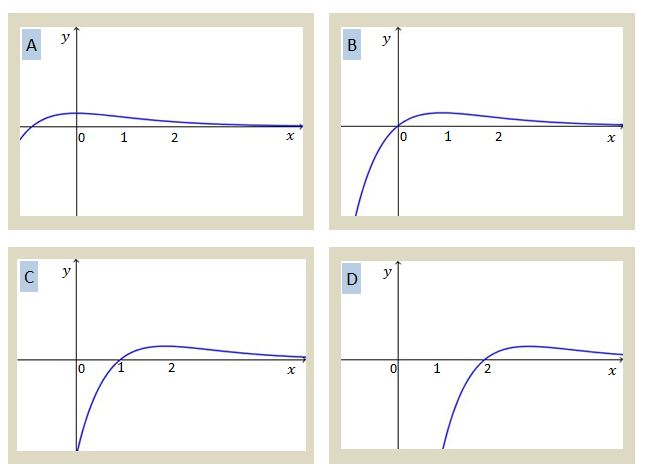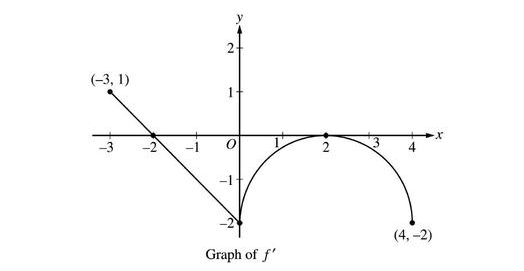Calculus

# Higher-order Derivatives: Level 2 ChallengesGiven the graph of $y=f(x)$ above, which of the following is a possible graph of $y=f''(x)?$Find the 2016-th derivative of $\sin ^{ -1 }{ (x) }$ at $x=0$.

###### Image Credit: Flickr Richard Stocker.

If a function $f(x)$ that is differentiable over $(-\infty,\infty)$ is monotonically decreasing and $\displaystyle\lim_{x\rightarrow\infty}f(x)\neq-\infty,$ then as $x$ approaches infinity, $f(x)$ is

$y = \tan^{-1}(x) , k! = \left. \dfrac { { d }^{ 21 }y }{ d{ x }^{ 21 } } \right|_{x=0}, \ \ \ \ \ k = \ ?$Suppose $f$ is a function defined on the closed interval $-3 \le x \le 4$ with $f(0)=42$ such that the graph of $f',$ the derivative of $f,$ on the interval is as shown in the above diagram. Find the $x$-coordinates of the points of inflection of $f.$

×